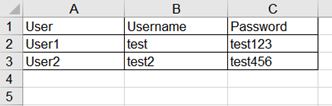June 6

## How to Read/ Write data from Excel for Selenium DataDriven tests in Python

### Introduction to Excel Data Driven Testing

To interact with excel in Python is a straightforward process. We have to use the openpyxl library in Python which helps to read/write in excel. This package is not available by default in the installed Python packages. We have to download by running the command pip install openpyxl from the terminal.

Conclusion: Thus we have discussed the openpyxl library in Python. For more details, you can refer to the link:

In the next section we shall discuss how to perform read/write operations with excel in Python.

### Operations to Read and Write the Data from Excel

Let us take an excel workbook having name PythonExcel.xlsx and populate the data within its Sheet1 as shown in the below image:To work with excel workbook in Python we have to add the import openpyxl package to our code. First of all we have to load the excel workbook with the help of the load_workbook method. The path of the excel workbook shall be passed as argument to the method. The load_workbook method is obtained from the openpyxl package.

Thus the book object has the location of the workbook. Next we shall need to know which sheet is active currently. We shall apply the active method on the book object and store it in the object variable named sheet.

sheet = book.active

Next to pinpoint to a cell in the sheet we shall take the help of the cell method. The row and column numbers shall be passed as arguments to the method.

cell = sheet.cell (row = 2, column = 1)

Here we are referring to a cell in the second row appearing in the first column. Finally to obtain the value out of that cell, we shall apply the value method on the cell object.

##### Code Implementation to perform read operation

import openpyxl

# to get active worksheet

sheet = book.active

# to pinpoint a cell at 2nd row and first column

cell = sheet.cell(row = 2, column = 1)

# to obtain the cell value

print(cell.value)

Now we shall discuss how to perform write operations. We have to access the cell on which we want to perform the write operation then set the value on to it.

sheet.cell (row = 1, column = 4).value = "Email", here we are setting the value Email in the cell appearing at the first row and fourth column.

##### Code Implementation to perform write operation

import openpyxl

# to get active worksheet

sheet = book.active

# set value with value method at 2nd row and 4th column

sheet.cell (row = 1, column = 4).value = "Email"

To get the maximum number of occupied rows in the active worksheet, we shall apply the max_row method on the active worksheet object. To get the maximum number of occupied columns in the active worksheet, we shall apply the max_column method on the active worksheet object.

rows = sheet.max_row

columns = sheet.max_column

Conclusion: Thus we have discussed how to interact with excel - read/write operations in Python. For more details, you can refer to the link:

In the next section, we shall discuss how to obtain values from an excel in Python.

### Build utility to retrieve values from sheet

To obtain all the data from the active worksheet, we have to iterate the rows from 1 to the sheet.max_row with the help of for loop. In the inner for loop, we have to iterate from 1 to the sheet.max_column. In this way, each of the occupied rows and columns get scanned.

##### Code Implementation to get all values in the excel

import openpyxl

# to get active worksheet

sheet = book.active

# to iterate through the rows

for i in range ( 1, sheet.max_row + 1):

# to iterate through the columns

for j in range ( 1, s.max_column + 1):

# to get the cell data and print

print (sheet.cell (row=i, column=j). value)

Conclusion

Thus we have discussed how to obtain values from an excel in Python. For more details, you can refer to the links:

In the next post, we shall discuss the Pytest framework.

Tags

## API Basics and Architecture

API Basics and Architecture

## Perform validations and execute tests in a workfow

Perform validations and execute tests in a workfow